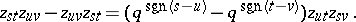# Quantum Grassmannian

A subalgebra $\mathcal{G} = \operatorname { Fun } _ { q } ( G ( k , n ) )$ in the algebra $\mathcal{A} = \operatorname { Fun } _ { q } ( \operatorname{SL} ( n , \mathbf{C} ) )$ of regular functions on the quantum group $\operatorname{SL} ( n , \mathbf{C} )$ (cf. Quantum groups). $\mathcal{G}$ is generated by quantum minors $| T _ { i _ { 1 } , \ldots , i _ { k } } ^ { 1 , \ldots , k } | _ { q }$, with $1 \leq i _ { 1 } < \ldots < i _ { k } \leq n$ and with $T \in \operatorname { Mat } ( n ) \otimes \mathcal{A}$ the vector co-representation of $\mathcal{A}$ [a1]. The $q$-minors satisfy quadratic relations, which turn into the Plücker relations (Young symmetries, cf. also Grassmann manifold) when the deformation parameter $q$ is specialized to $1$. Since classically the Grassmannian, as a complex submanifold in the projective space $\mathbf{P} ( \wedge ^ { k } \mathbf{C} ^ { n } )$, is the common zero locus of the Plücker relations, one interprets $\mathcal{G}$ as a quantization of the complex Poisson manifold $G ( k , n )$ (cf. Symplectic structure). The co-multiplication $\Delta$ in $\mathcal{A}$ induces a right co-action $R = \Delta |_{\cal G} :\cal G \rightarrow G \otimes A$ and so $\mathcal{G}$ is a quantum homogeneous space.

A more general construction of (generalized) quantum flag manifolds exists for the group $\operatorname{SL} ( n , \mathbf{C} )$ [a1], as well as for other simple complex Lie groups $G$ having quantum counterparts [a2]. Another description was given in [a3]. Both approaches [a2], [a3] also allow one to define quantum Schubert varieties.

Since $G ( k , n )$ is compact, the only holomorphic functions defined globally on it are the constants. But one can work instead with holomorphic coordinates $z _ { s t }$, $1 \leq s \leq k$, $1 \leq t \leq n - k$, on the big cell $C$, the unique Schubert cell of top dimension. The standard choice of coordinates is given via the Gauss decomposition of $T$. For the algebra $\mathcal{G}$ this means in fact a localization by allowing the $q$-minor $| T _ { 1, \dots, k } ^ { 1 , \ldots , k } | _ { q }$ to be invertible. The generators $z _ { s t }$ of the quantum big cell $\mathcal{C} = \operatorname { Fun } _ { q } ( C )$ satisfy the relations [a4]The symplectic manifold $G ( k , n )$ can be realized as an orbit of the dressing transformation of $\operatorname{SU} ( n )$ acting on its dual Poisson Lie group. The transformation can be also viewed as the right $\operatorname{SU} ( n )$-action on the manifold $M$ of $( n \times n )$ unimodular positive matrices: $( m , u ) \mapsto u ^ { * } m u$. The orbits are determined by sets of eigenvalues and $G ( k , n )$ corresponds to a two-point set $\{ \lambda _ { 1 } , \lambda _ { 2 } \}$ with multiplicities $k$ and $n - k$, respectively. There exists a quantum analogue as a right co-action

\begin{equation*} \operatorname { Fun } _ { q } ( M ) \rightarrow \operatorname { Fun } _ { q } ( M ) \bigotimes \operatorname { Fun } _ { q } ( \operatorname {SU} ( n ) ). \end{equation*}

$\operatorname { Fun } _ { q } ( M )$ is endowed with a $*$-involution and, correspondingly, one can turn $\mathcal{C}$ into a $*$-algebra by determining the commutation relations between $z _ { s t }$ and $z ^ { * _{ u v}}$ in dependence on the parameters $\lambda _ { 1 }$ and $\lambda _ { 2 }$ [a4].

Similarly as for quantum spheres (cf. Quantum sphere), other types of quantum Grassmannians have been defined, distinguished by possessing classical points, i.e., one-dimensional representations $\operatorname {Fun}_q ( G ( k , n ) ) \rightarrow \mathbf C$ [a5].

How to Cite This Entry:
Quantum Grassmannian. Encyclopedia of Mathematics. URL: http://encyclopediaofmath.org/index.php?title=Quantum_Grassmannian&oldid=50656
This article was adapted from an original article by Pavel Å tovÃ­Äek (originator), which appeared in Encyclopedia of Mathematics - ISBN 1402006098. See original article